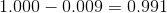## Example Questions

### Example Question #2 : Problem Solving

A runner runs 10.2 miles east, then 2.3 miles west, then 1.4 miles east.

How many miles did the runner travel from where he started? (How far east did the runner go)?

6.5 miles

19.3 miles

9.3 miles

10.2 miles

13.9 miles

9.3 miles

Explanation:

When the runner is travelling east, it's in the positive direction. West is the negative direction. So we can compute it by doing the operation 10.2 + (–2.3) + 1.4 = 9.3

### Example Question #4 : Problem Solving

What is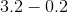?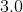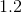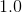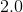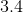Explanation: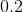removed from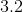results in the whole integer.

### Example Question #5 : Problem Solving

Subtract: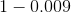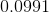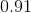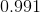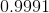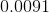Rewriteadding the correct number of placeholders and evaluate.